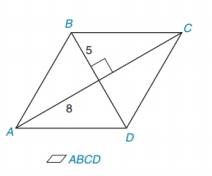Chapter 8.2, Problem 15E### Elementary Geometry for College St...

6th Edition
Daniel C. Alexander + 1 other
ISBN: 9781285195698

#### Solutions

Chapter
Section### Elementary Geometry for College St...

6th Edition
Daniel C. Alexander + 1 other
ISBN: 9781285195698
Textbook Problem
3 views

# In Exercises 13 to 18, find the area of the given polygon.To determine

To Find:

Area of the rhombus.

Explanation

Formula Used:

The area A of a rhombus whose diagonals has lengths d1 and d2 is given by A=12d1d2.

Diagonals of a rhombus are perpendicular bisector of each other.

Calculation:

It is given that AB¯=BC¯. Let the diagonals meet at O.

Also given that BO=5 and AO=8.

In rhombus, opposite sides are parallel and all sides are in equal length.

Therefore, AO=OC=8 and BO=OD=5

### Still sussing out bartleby?

Check out a sample textbook solution.

See a sample solution

#### The Solution to Your Study Problems

Bartleby provides explanations to thousands of textbook problems written by our experts, many with advanced degrees!

Get Started